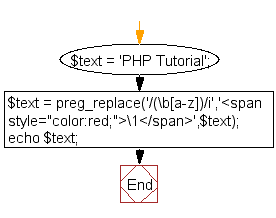﻿ PHP Exercise: Changes the color of first character of a word - w3resource

# PHP Exercises: Changes the color of first character of a word

## PHP: Exercise-9 with Solution

Write a PHP script, which changes the color of the first character of a word.

Sample string : PHP Tutorial

Sample Solution: -

PHP Code:

``````<?php
\$text = 'PHP Tutorial';
\$text = preg_replace('/(\b[a-z])/i','<span style="color:red;">\1</span>',\$text);
echo \$text;
?>
``````

Sample Output:

`PHP Tutorial`

View the output in the browser

preg_replace() function:

Syntax:

`mixed preg_replace ( mixed \$pattern , mixed \$replacement , mixed \$subject [, int \$limit = -1 [, int &\$count ]] )`

Searches subject for matches to pattern and replaces them with replacement. The function returns an array if the subject parameter is an array, or a string otherwise. If matches are found, the new subject will be returned, otherwise subject will be returned unchanged or NULL if an error occurred.

Flowchart:Have another way to solve this solution? Contribute your code (and comments) through Disqus.

What is the difficulty level of this exercise?

Test your Programming skills with w3resource's quiz.

﻿

## PHP: Tips of the Day

Mutates the original array to filter out the values specified

Example:

```<?php
function tips_pull(&\$items, ...\$params)
{
\$items = array_values(array_diff(\$items, \$params));
return \$items;
}

\$items = ['x', 'y', 'z', 'x', 'y', 'z'];
print_r(tips_pull(\$items, 'y', 'z'));
?>
```

Output:

```Array
(
 => x
 => x
)
```